# Lecture 24 - Quasars and Active Galaxies (4/15/99)Expansion of the Universe --- | --- Cosmology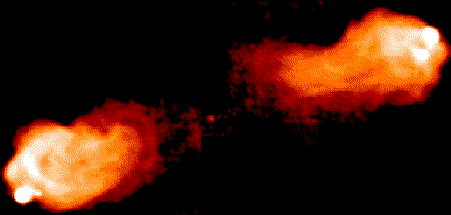Reading:
Chapter 23, 24 (ZG4)

Notes:
pages 96-101

The radio galaxy Cygnus-A (M87) as seen by the VLA at 20cm. (Courtesy Phil Blanco/UCSD)
Key Question: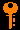## Investigations:

1. Redshift
• Why does the wavelength of light expand with the Universe?
• How is the redshift z of a galaxy related to the Doppler shift?
• What is the characteristic distance c/H, or Hubble distance, associated with the expansion?
• What is the characteristic timescale 1/H, or Hubble time, associated with the expansion?
• What does H=60 km/s/Mpc imply for the age and scale of the Universe?
• What is a peculiar velocity and how does it affect the use of the Hubble relation as a distance indicator?
• How can we measure a peculiar velocity?
• If the expansion velocity v is constant, what is the significance of the timescale 1/H?
• If the Hubble constant H is constant, what is the form for the expansion x(t)?
• Why is the current value of the Hubble constant, H_0, used instead of just H?
• What do we mean by the Big Bang, and why need there not be an explosion or center to account for an expanding universe?
• How is the redshift z related to the scale factor a of the Universe, where r(z=0) = a*r(z) for some physical distance r?
2. The Universe at High Redshift (z > 0.1)
• What is the distance modulus for the Hubble distance (H=60) of 5000 Mpc?
• How bright would a supernove (M = -20) be at this distance?
• How bright would a bright cluster galaxy (M = -23) be?
• Is this brightness level easy to obtain?
• Why do some galaxies have very bright nuclei?
• Why are some galaxies unusually bright in the radio?
• Why do some galaxies have strong emission lines?
• Why do some galaxies show extreme star formation rates?
• When did radio astronomy develop?
• What did Karl Jansky discover for the source of low-frequency (167 MHz) celestial radio emission?
• What sorts of radio sources did Grote Reber find when he pointed his backyard radio telescope skyward in 1936?
• What wartime deveopments allowed radio astronomy to leap forward after WWII?
• Why is synchrotron emission strong in our galaxy?
• Why are supernova remnants bright in radio emission?
• Why do some galaxies have huge radio luminosities?
• Why are radio galaxies often referred to as classical radio doubles ?
• What parts of a radio galaxy do core, jet, hot spot, and lobe refer to?
• Where is the optically identified galaxy located with respect to the radio emission?
• At what rate must mass be converted to energy (E=mc2) to supply the observed power of 1038 Watts for Cygnus-A?
• How much total mass has been converted to produce the total energy of 1052 J? What lifetime does this imply?
• Why does the morphology suggest that the energy is generated in the center of the galaxy and transported to the lobes by the relativistic jets?
• Why do we know there are relativistic electrons involved?
• Why do we believe that supermassive black holes are involved?
• At what rate must stars be devoured to supply the energy needs of Cygnus-A?
5. Quasars
• Why do some double radio sources appear to have "quasi-stellar objects" at their centers and not galaxies?
• What does the term quasar mean?
• What did Maarten Schmidt discover in 1962 when he finally identified the mysterious absorption lines in 3C273 and 3C48?
• What is the absolute magnitude of 3C273 if it has m_v = 13 and is at a redshift z = 0.16 (d = 800 Mpc)? Why is this surprising?
• What is the likely source of this emission, given that it is observed to originiate within a parsec or so of the core?
• What is the mass of the black hole needed to supply the luminosity of 1013 Lsun at the Eddington luminosity?
6. Central Engine: Supermassive Black Holes
• How do you build a 108 Msun black hole at the center of a galaxy?
• Why might the jet axis be aligned with an angular momentum axis of an accretion disk?
• Why do we expect magnetic fields to be involved?
• What is the Schwarzschild radius of a 108 Msun black hole?
• What magnetic field strengths are needed near a black hole to supply the Blandford-Znajek power

P ~ B2 RS2 c

• What is the energy density for a magnetic field of B gauss? Why do we use cgs units here?
• What do we mean by equipartition of magnetic and particle energy?
• What magnetic field strengths are implied by an energy of 1059 ergs in a radius of 9 kpc?
• How does the relativistic jet carry momentum? What is the rate of momentum transfer for jet power P?
• If the hot-spot area is pi*R^2, and the IGM density is rho, then what is the advance speed implied for R=9 kpc and P=10^38 W, if n ~ 1/m^3 in the IGM?
• What is the time needed to advance 50 kpc at these speeds, and how does it compare to our luminosity lifetime?
• How does our supermassive black hole picture fit together will all these parts?
7. Gravitational Lenses
• Why is light bent near a black hole? Should it be bent near other masses?
• Why is the characteristic scale for lensing the Schwarzschild radius? What is the Schwarzschild radius for a 10^12 Msun galaxy?
• How many R_S are needed to deflect light by 1"?
• Why are point sources behind galaxies "imaged" into multiple (2 or 4) magnified images?
• Why are background extended objects lensed into Einstein rings?
• What does lensing tell us about the mass of the lensing galaxy and the scale of the lens? How can this be a distance indicator?
• Do clusters of galaxies lens background galaxies also?
8. Large Scale Distribution of Galaxies
• Are galaxies uniformly distributed about the universe?
• What are clusters of galaxies?
• What are the members of the Local Group of galaxies?
• How do we get the 3rd dimension (distance) in our maps of large-scale structure?
• Why do clusters look "big" along the redshift dimension (ie. "finger of God")?
• What are voids in the galaxy distribution?
• What are walls and filaments?
• What is a supercluster?
• What does the clustering of galaxies tell us about the universe?
9. Clusters of Galaxies
• Are clusters like Virgo or Coma gravitationally bound objects?
• How is the velocity dispersion calculated?
• How are the 3-D and radial velocity dispersions related?
• What is the binding energy of a uniform density spherical cluster of radius R and mass M?
• What does the virial theorem tell us the velocity dispersion is of this cluster?
• Given the radial velocity dispersion what is the virial mass of a cluster?
• What is the virial temperature of gas in a cluster of R=1.5 Mpc and M=10^15 Msun?
• Why is this gas bright at X-ray wavelengths?

## Large Scale Structure of the Universe in Outline

1. Active Galactic Nuclei
• Some galaxies have extremely luminous nuclei, often outshining the rest of the galaxy.
• These objects are called active galactic nuclei. The Milky Way appears to have such an active nucleus.
• Some active galaxies, like our Milky Way, appear to be powered by supermassive black holes at the center.
• Other types of active galaxies show evidence of widespread star formation in the inner hundreds of parsecs.
• Some of these star-forming galaxies, called starbursts, can also be very luminous from large numbers of O and B stars and H II regions.
• Supernovae in the starburst regions will also produce large amounts of energy.
• Many of the brightest radio sources are associated with distant galaxies.
• The first crude maps showed a "double" structure to these radio galaxies.
• The radio emission comes from a region much larger than the optical galaxy - often greater than 1 Mpc in extent.
• The emission is synchrotron radiation from relativistic electrons moving in weak magnetic fields.
• This jets of emission extend from a central nucleus to hotspots at the far edges of the lobes.
• The jets feeding the electrons outward to the lobes from the central black hole (or other energy source).
• The total radio luminosity from synchrotron in powerful radio galaxies is 10^36 to 10^38 Watts (10^10 to 10^12 Lsun).
• The total energy contained in the lobes is about 10^53 J, equivalent to 10^6 Msun c^2 of mass-energy! This could be obtained over the typical 10^6 year lifetime of a radio source by conversion of 1 Msun per year into energy.
• The likely source for this energy is a supermassive black hole of mass 10^6 to 10^8 Msun. Such a monster could gravitationally extract 10% of the rest mass energy of 10 Msun/yr of fuel to supply the needed power.
• Some of the largest radio galaxies extend out to several Mpc on either side of the nucleus.
• Some radio sources show evidence of interaction with intergalactic gas, being swept backward as the galaxy moves forward at a few hundred km/s.
3. Quasars
• Some bright radio sources seemed to be not associated with galaxies but with stellar like objects.
• These are quasars, or "quasi-stellar radio sources".
• The optical spectra of quasars showed strange lines which did not seem to correspond to known elements.
• Maarten Schmidt at Palomar Observatory realized in 1962 that these were highly redshifted Balmer lines of hydrogen!
• This was controversial since these redshifts (z=0.16 for 3C273 and z=0.37 for 3C48, the two first discovered) indicated extreme distances and thus huge luminosities (10^15 Lsun).
• Quasars were soon found with redshifts z > 1. These were at that time the most distant objects known.
• Currently, the most distant quasar known has a redshift z > 5!
• Some quasars are were discovered that did not have any radio emission - these were dubbed QSOs, or "quasi-stellar objects".
• Some of the quasar emission may be beamed, like a searchlight.
4. Gravitational Lenses
• For lensing by a galaxy (10^12 Msun) instead of a star (1 Msun), the splitting are an arcsecond or more - easily seen!
• The first lens, a double quasar, was found in 1979.
• Currently, there are over 30 gravitational lenses known.
• There are a number of gravitational lens surveys being carried out that will greatly increase the number of known lenses in the next few years.
• Gravitational lenses magnify the background galaxy or QSO, acting as a gravitational telescope.
• The different light ray paths that correspond to the different images have different lenghts, so there are relative time delays that can be seen if the source is variable.
• If the time delays can be measured in addition to the image separations and relative magnifications, then the distance to the lens and source can be separated out from the mass of the lens.
• This will lead to a measurement of the Hubble constant independent of the standard distance ladder.
• Clusters of galaxies (10^15 Msun) can also act as gravitational lenses, distorting and magnifiying background galaxies and QSOs. The image separations are larger (>10") and usually result in arcs as extended background galaxies are smeared out and highly magnified.
• Cluster lenses are an important tool for measuring the mass in clusters.
5. Luminosity Function of Galaxies
• What is a luminosity function? How does it describe the number density of galaxies of a given luminosity per Mpc^3?
• What is the Schechter luminosity function?
• What is meant by a L* galaxy?
• At low luminosities, why does the Schechter function predict a diverging (infinite) number of faint galaxies, yet a finite total luminosity density?
• Why do we measure distances in the units of h^-1 Mpc, where H0 = 100 h km/s/Mpc?
• About how many galaxies of L* or brighter are there per cubic Mpc?
6. Large Scale Distribution of Galaxies
• Are galaxies uniformly distributed about the universe?
• What are clusters of galaxies?
• What are the members of the Local Group of galaxies?
• How do we get the 3rd dimension (distance) in our maps of large-scale structure?
• Why do clusters look "big" along the redshift dimension (ie. "finger of God")?
• What are voids in the galaxy distribution?
• What are walls and filaments?
• What is a supercluster?
• What does the clustering of galaxies tell us about the universe?
7. Clusters of Galaxies
• Are clusters like Virgo or Coma gravitationally bound objects?
• How is the velocity dispersion calculated?
• How are the 3-D and radial velocity dispersions related?
• What is the binding energy of a uniform density spherical cluster of radius R and mass M?
• What does the virial theorem tell us the velocity dispersion is of this cluster?
• Given the radial velocity dispersion what is the virial mass of a cluster?
• What is the virial temperature of gas in a cluster of R=1.5 Mpc and M=10^15 Msun?
• Why is this gas bright at X-ray wavelengths?

## Interacting Galaxies

Computer simulations of the interactions between galaxies have been an important development in our understanding of this process. In essence, model galaxies are set up with "stars" (particles) and "gas" (fluid elements), and then moved along a grid interacting via Newtons laws and hydrodynamics. Early simulations demonstrated the production of "tidal tails". Current state-of-the art simulations are showing the extent of the influence of interactions on the evolution of galaxies in our universe.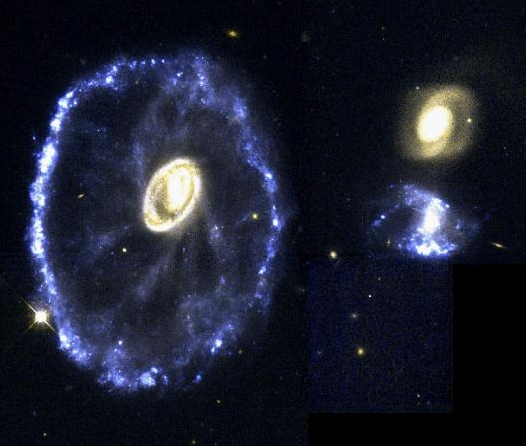The Cartwheel Galaxy, courtesy HST/STSCI

The above image is from the Hubble Space Telescope, and shows a galaxy that has been disturbed by what was probably a direct hit by the (also disturbed) small blue galaxy on the right. The ring and spokes are what is left of the disks of the galaxies, with shock-induced star formation going on in the ring, and some gas accumulating in the center of the remnant.

Some galaxy interaction resources:

## Supermassive Black Holes at the Centers of Galaxies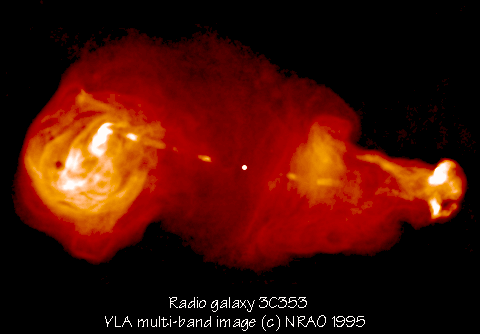Radio galaxy 3C353 multi-band image from VLA, courtesy Alan Bridle (NRAO).

## Gravitational Lenses

One reason we believe that quasars really are very distant is because sometimes we see them lensed by a distant galaxy between us and the quasar!

A galaxy in front of a background galaxy or quasar will gravitationally lens the background source by the bending of the light rays. Since galaxies are 10^12 times more massive than stars, the image splittings are arcseconds instead of 10^-6 arcseconds (angle proportional to the square-root of the mass).

I am currently involved in the Cosmic Lens All-Sky Survey (CLASS) which is currently mapping around 10000 radio sources looking for indicences of multiple images indicative of lensing. So far, we have found 5 new lenses and a large number of candidates that we are checking whether they are lenses. For a description of CLASS and the lenses we have found, see my CLASS Home Page.

Large clusters of galaxies, which contain thousands of galaxies and total masses of 10^14 to 10^15 Msun within a radius of several Mpc, also act as powerful gravitational lenses. Background galaxies and QSOs are magnified and distorted into arcs by the lensing action of the cluster. The Hubble Space Telescope has taken some spectacular pictures of gravitational lensing by the cluster Abell 2218For more on the gravitational lenses I found in my VLA-based radio survey, see the Penn CLASS Page.

## Large Scale Surveys of Galaxies

There are a number of large-scale surveys aimed at measuring redshifts of a large number of galaxies and thus delineating the structure that we see in the Universe around us.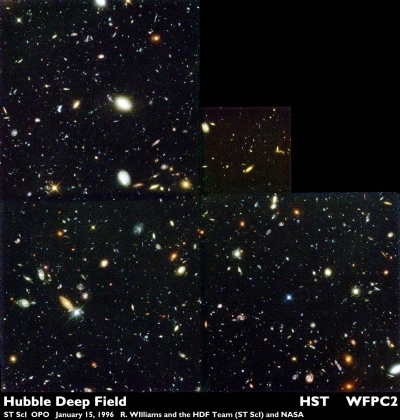The Hubble Deep Field. Courtesy HST/STSCI

Though not a redshift survey, the Hubble Deep Field (HDF) is also revolutionising our understanding of the distant universe, as we can see galaxies as they were during the early phases of life.Prev Lecture ---Next Lecture ---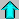Astr12 Index ---Astr12 Home

smyers@nrao.edu Steven T. Myers